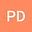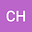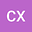On the model of equatorial Magnetic-Archimedes-Coriolis waves propagating at Earth's core surface and the potential implications
•••Cancan Xu
Shanghai Astronomical Observatory
Author Profile## Abstract

The purposes of this work are (1) clarifying the specific latitude range in which the currently physical model of the equatorial trapped Magnetic-Archimedes-Coriolis (namely eMAC) waves propagating atop the Earth’s core can own the enough accuracy to describe the hydromagnetic waves; (2) presenting the systematically analytical expressions to represent the physical properties (e.g., the equatorial confinement and latitudinal distribution, damping rate α, eigen-period T) of the eMAC waves. Here, the new results indicate that: 1) the eMAC wave model can own the high accuracy (i.e., the relative errors are less than 5%) to describe the core waves in the regions with latitude below 25 degrees ; 2) the equatorial confinement and latitudinal distribution law is essentially governed by a specific solution form with the typical Hermite polynomial term of degree n; 3) the damping rate can be estimated by α ≈-π^2/(μσH^2) (μ being the vacuum permeability, σ being the core electrical conductivity; H being the stratified layer thickness of the core), showing that the magnetic diffusivity η (=1/(μσ))can cause the ohmic dissipation of the waves; besides, the H value is predicted to be larger than 20km, when T matches the observed 8.5yr period. This work also presents the analytical models for the perturbed magnetic fields due to the eMAC waves, presenting that the azimuthal perturbed magnetic field bφ(with degree n=1) is mainly confined to the equatorial regions with latitude below ~15 degrees, the profile of which coincides with the observed core surface azimuthal flows.